## Boost Converter

Boost Converter is a power converter and found in many applications where input voltage is not sufficient to drive the application.Boost Converter Assembled Circuit
Let see more details about boost converter;

### What is Boost Converter?

• A boost converter is a type of DC-to-DC power converter that steps up the applied input voltage and steps down the current.
• In other words, we can say that a boost converter is a DC to DC converter with an output voltage higher than the applied input voltage.
• A boost converter sometimes also called as “step-up converter” as it "steps up" the applied input voltage.
• Here the output current is lower than the applied source current to save power or to get more efficiency.
• It is a type of switched-mode power supply which contains a diode, a FET, an inductor and an electrolytic capacitor.

### Need of Boost Converter

• Switched mode power supplies can be used for many types of applications such as; DC - DC converters.
• Many times to run the motors, to operate tools, to charge the battery bank, etc. we need high DC voltage.
• But mostly the available DC voltage (mostly from battery) is not sufficient to run these applications we need a higher DC voltage as compare to applied DC input voltage.
• To run the high power applications, we can use many batteries by connecting in series and parallel combination, but this solution will consume more space and will also increase the cost.
• By using the Boost Converter, we can boost the battery voltage, AC- DC rectifier voltage, DC generators voltage, etc. to required high voltage.
• But with the increase in output voltage there is decrease in output current.

### Boost Converter Circuit

Figure at below shows the basic circuit of a Boost converter.Boost Converter
• In this we can see that a MOSFET M1 is used as switching device, an inductor L1 is used for storing the energy, Diode D1 which conducts when it is forward bias and at last an Electrolytic capacitor C1 is used to store the charge.
• We can see that the used components are same but their placement in the circuit has been rearranged.

### Working of Boost Converter

Below images and explanations clears the working of boost converter;

#### Working of Boost Converter when switch is ONWorking of Boost Converter when switch is ON
• In above figure we can see that a pulse input is applied to the MOSFET. When high voltage is available at MOSFET gate, it starts to conduct.
• As MOSFET conducts, it will make current to pass from drain to source terminal of MOSFET and hence inductor L1 start to stores the energy as the current passes through it, i.e. from power supply’s +ve to -ve terminal.
• Inductor L1 stores energy in the form of the magnetic field.
• No current passing through the diode D1, capacitor C1 and through the load which have high impedance as compare to the MOSFET’s drain to source path.

#### Working of Boost Converter when switch is OFFWorking of Boost Converter when switch is OFF
• In above figure we can see that when low pulse voltage is available at MOSFET gate, it didn’t conduct.
• As MOSFET not conducts, it will make current not to pass from drain to source terminal of MOSFET.
• Because of MOSFET’s fast ON and OFF switching a sudden drop in current will appear, which will generate a back e.m.f. in opposite direction in inductor L1.
• So, two voltages will appear across inductor L1. First is applied input voltage and second is back e.m.f.
• Now inductor starts to pass the current through forward biased diode D1.
• This current charges further the electrolytic capacitor C1 and further it is applied to the connected load.
• Capacitor C1 will charge with the voltage, “Input voltage + back e.m.f. voltage”.

#### Working of Boost Converter when switch is again ONWorking of Boost Converter when switch is again ON
• In above figure we can see that when again high pulse voltage is available at MOSFET gate, it starts to conduct.
• When MOSFET is ON the D1 acts as reverse biased i.e. cathode of D1 diode is more positive, because it is connected to the capacitor C1 and it is already fully charged.
• As the diode D1 is not conducting, the load at output will get voltage from capacitor C1, until it discharges.
• Capacitor fully drains and it will recharge again when the MOSFET M1 switch OFF again.
• That’s why we get constant output voltage across the load.

### Modes of operation of Boost converter

There are three modes of operation of boost converter;

#### Continuous conduction mode (CCM)

In this mode of boost converter mode, the current flowing through inductor never goes to zero. In other words, we can say that the inductor partly discharges the current before the start of the new switching cycle.

#### Discontinuous conduction mode (DCM)

In this mode of boost converter, the current flowing through inductor goes to zero. In other words, we can say that the inductor is fully discharged at the end of present switching cycle.

#### Critical conduction mode (CRM)

The critical conduction mode works in the boundary of CCM and DCM.

Please refer below link of our previous article to get more details about modes of operation of boost converter;

"Power Factor Correction and its modes of operation"

### Boost Converter IC UC3854

UC3854 is High Power Factor Preregulator and widely used in the power factor correction applications i.e. for active PFC. Where it controls switching of MOSFET to get high voltage at the output.

Please refer our previous articles to get more details about boost converter design, boost converter calculations and boost converter schematic using UC3854;

"Design of Boost Converter using UC3854"

### Boost Converter IC L6562

The L6562 is a transition mode PFC controller and widely used in power factor correction and lighting applications.

Please refer our previous article to get more details about boost converter calculations using L6562;

"Critical Conduction Mode Boost Converter Calculations using L6562"

### Application of Boost Converter

• In most of DC power supplies they are used to boost low voltage to high voltage.
• In lighting applications to drive LED lamps, Fluorescent lamps and Halogen lamps.
• In automobile application for battery charging.
• In charging of battery bank.
• It can be seen in regenerative braking of DC motors.
• In Power Factor Correction

### Conclusion

Boost converter is widely used power converter topology. We can use it in the applications where available input voltage is not sufficient to drive the circuit properly. With the implementation of boost converter, we can achieve high voltage at the output but also it decreases the output current because the output wattage is constant.

## Buck-Boost Converter

### What is Buck-Boost Converter?

• We can define Buck-Boost Converter as a type of DC – DC converter whose generated output voltage that is either higher or smaller than the applied input voltage.
• Buck-Boost Converter is alike a flyback converter, but difference is that it uses inductor and flyback converter uses transformer.
• Buck-Boost Converter is a combination of Buck Converter and Boost Converter.
Below figure shows a Buck-Boost Converter Module;

To know more about Buck Converter please visit the article, "What is Buck Converter".

There are two different topologies of buck-boost converter. Inverting topology and non-inverting topology. In detail both are explained below;

### The inverting topology

• Like buck converter and boost converter the inverting buck-boost converter is like a switched-mode power supply and its circuit topology is same like buck converter and boost converter.
• The polarity of the output voltage is opposite as compare to the applied input voltage.
• For simple or basic inverting buck-boost converter we can see or get a negative output voltage with respect to the ground.
• The duty cycle of the switching transistor or MOSFET, the output voltage of buck-boost converter is adjustable or changeable.
• The driving circuitry becomes complicate, when we see that the switching component does not have a terminal at ground. Which can be a disadvantage.
• But, this disadvantage is of no importance. If the power supply is isolated from the load circuit because at this condition the power supply and polarity of diode can be upturned or reversed.
• At the reversed condition, the switch can be on either the supply side or at the ground side.

### Working of inverting topology

• An Inverting buck-boost converter is shown in below figure. It is the schematic of a basic inverting buck-boost converter which is working in continuous conduction mode (CCM).
• We can see an output capacitor, in the power stage a metal-oxide semiconductor field-effect transistor (MOSFET) is present, a diode, and an inductor is present.
• Anode of the diode is connected to the load.
• Anode of the diode is also connected to negative terminal of electrolytic capacitor.
• When the MOSFET (M1) is ON, the voltage through the inductor (L1) is Vin, and the current in the inductor ramps up with the rate that is proportional to the applied input voltage Vin.
• This results in storing energy in the inductor L1. While M1 is ON, the output capacitor C1 supplies the entire load current.
• When the M1 is OFF, the diode (D1) is forward-biased and the inductor current ramps down at a rate proportional to output voltage Vout.
• While M1 is OFF, energy is transferred from the inductor L1 to the output load and output capacitor C1.

### The non-inverting topology

• Below figure shows a non-inverting buck-boost converter. Here we can see a buck (step-down) converter is joint with a boost (step-up) converter.
• The output polarity of this type of converter is same like the polarity of the applied input. But the output level may vary i.e. may be higher or lower depending on the design and component selection.
• We can see in the figure that there is only one inductor is used even there is buck mode and boost mode is combined.
• This single inductor controlled by switches instead of diodes. At a time only one switch is in ON condition.
• If it uses many inductors with only one switch same like seen in Cuk or SEPIC topologies, then it is called as "four-switch buck-boost converter".
• The popular non-inverting buck-boost topologies are; Zeta, SEPIC and two-switch buck-boost converter.
• All these above mentioned buck boost topologies generate positive output but these topologies have extra power components and less efficiency as compared to a basic inverting buck-boost converter.

### Working of non-inverting topology

• We can see in figure of non-inverting buck-boost conductor; two high frequency switching MOSFETS are used along with the two diodes and these diodes have a low forward junction voltage when it conducts.

### Operation of buck converter

• We can understand the operation of buck-boost inductor on the basis of inductor's "reluctance", which allows quick change in current (current across inductor).
• Initially current through the inductor is zero. This means at the initial stage when MOSFET is not working i.e. switch is open and there’s no current across the inductor i.e. nothing is charged.
• When the MOSFET switch is first closed, the blocking diode stops current from flowing through it as it is reversed biased, so the current passes through the inductor.
• Initially the Inductor will keep the current low by dropping most of the source voltage, as the inductor doesn't like fast current change. With the time the inductor allows the current to increase slowly with the decrease in voltage drop. By this inductor will store energy i.e. magnetic field.
Let see the operation of buck converter in step wise in detail;

• Below figure shows the mode of operation of buck converter when MOSFET M1 is ON. In this mode the high frequency square wave generated by IC keep the MOSFET M1 ON and OFF. MOSFET M2 is turned off in this case.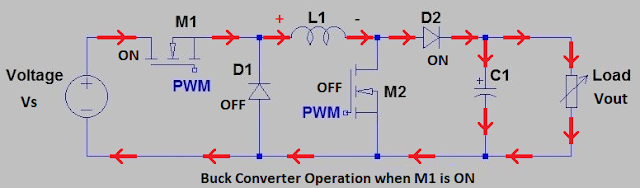Buck converter operation when M1 is ON
• When the gate terminal of MOSFET M1 is high, M1 starts to work and makes current to flow though inductor L1. This current charges L1, capacitor C1 and supply further to the connected load.
• The diode D1 is turned off because of the positive voltage on its cathode i.e. it is reversed biased.
• Below figure shows the mode of operation of buck converter i.e. current flow when MOSFET M1 is OFF. Now the inductor L1 is fully charged and now it is the only source of the current.Buck converter operation when M1 is OFF
• The magnetic field generated across the inductor L1 starts to collapse and generates back E.M.F. this E.M.F. opposites the voltage polarity across L1.
• This polarity change turns on the diode D1 and current flows through the diode D2 and further to the connected load.
• Further as the current across inductor L1 decreases, the charge stored in capacitor C1 during the ON time of MOSFET M1, now adds to the current flowing across the load. This additional current from C1 keeps output voltage (Vout) constant during the M1 OFF time.
• This gives an advantage of less ripple at the output.

### Operation as a Boost Converter

• Below figure shows the mode of operation of boost converter when MOSFET M1 is continuous ON. In this mode the high frequency square wave generated by IC is applied to the MOSFET M2 gate terminal.Boost converter operation when M2 is ON
• When MOSFET M2 is ON and it’s conducting, the input current passes across the inductor L1 and through M2, and further current flow to the negative terminal of supply. This current charges or creates magnetic field around inductor L1.
• Also in this step the diode D2 not conducts i.e. current not flows across the diode D2 because high speed conducting MOSFET M2 keeps anode terminal of diode D2 at ground potential.
• Now for this ON period, the charge developed by previous oscillating cycles on the capacitor C1 acts as a supply for the load.
• The slow discharge of capacitor C1 throughout the ON period and its immediate recharging creates high frequency ripple on the output voltage.
• This high frequency ripple is at a potential of approx. Vs + Vout.
• OFF-Period of MOSFET M2 can be understand by below figure;
• As the OFF-period of MOSFET M2 starts, the inductor L1 charges and capacitor C1 partially discharges.
• As the inductor L1 charged it generates a back E.M.F. The value of this E.M.F. depends on the rate of change of current as MOSFET M2 switches OFF and ON the total inductance. This inductance is hold by the coil (inductor).
• So, depending on the circuit design the back E.M.F. can be of any voltage range.
• Now the polarity of the voltage across inductor L1 has now reversed and this voltage is added to the input voltage Vs. This gives an output voltage that is equal or greater than the applied input voltage Vs.
• Diode D2 is now forward biased i.e. it starts to conduct and so the current further flows through the load.
• At the same time this current from diode D2 re-charges the capacitor C1. Which will be further use in next period of MOSFET M2 ON.

### Applications of Buck-Boost Converter

• We can see Buck-boost (step-down and step-up) converters mostly in the applications like; most of industrial machines or tools where different power supply voltages are needed for machine or tool operation, industrial computer hardware’s and automotive systems.
• Battery dependent systems; where at the full charge of battery, the high voltage can’t be supply to the system, then buck regulator circuit works. But as the battery charge comes to end the boost regulator circuit starts to work and boost the available voltage to the required level, so the system works properly.
• These types of battery depended systems can be seen in automotive applications i.e. mostly in e-bikes or e-cars.
• In the applications mentioned above, the applied input voltage could be either higher or lower than the required output voltage.

### Conclusion

By joining these two regulator designs i.e. buck regulator and boost regulator we can have a regulator circuit which can handle a wide range of input voltages. These input voltages may be both higher or lower than that required by the circuit.

Both buck and boost regulator uses same types of components and we have to just re-arrange them, depending on the applied input voltage level.

## Ohm's law Calculations and Calculator

Ohm’s law many of us heard many times. To understand ohm’s law is very important for electrical and electronics engineer, technicians, hobbyist, etc. Here we will discuss in detail about Ohm’s law definition, Ohm’s law calculations and calculator.Ohm's Law

### Ohm’s law Calculator

Enter any two known values and press "Calculate" to get solutions of the others.
Fields should be reset to 0 or clear before each new calculation.
Volts
Amps
Ohms
Watts

This Ohm’s law calculator will help you to perform ohm’s law calculations easily. Even manually calculated values using ohm’s law formula you can compare with the results of this calculator.

### Ohm’s law Calculations and Calculator Excel Sheet

An excel sheet for Ohm's law calculation you can access by clicking on below button. Download it and save in your laptop, computer, tablet or mobile;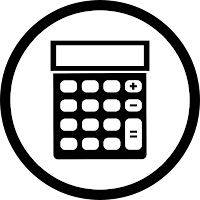Ohm's law Calculator
Now let discuss Ohm’s law definition and its calculation in detail;

### What is Ohm’s law

Definition of Ohm’s law is, “the current through a conductor between two points is directly proportional to the voltage across the two points”.

Introduce Resistance as constant of proportionality. Resistance in below equation is constant and not dependent of the current.

The German physicist Georg Ohm invented this law. As per him, at a constant temperature, the current flowing through a fixed linear resistance is directly proportional to the voltage applied across it, and inversely proportional to the resistance.

In other words, we can say that, the relationship between Voltage, Current and Resistance is called as Ohm’s law.

Ohm's law is an empirical relation i.e. experimental relation which precisely defines the conductivity of the many of electrically conductive materials over different level of current.

Non-ohmic material do not follow Ohm's law.

### Ohm’s law equation

The mathematical equation for the same can be given as; Resistance = Voltage / Current.
Where,
Current (I) = current through the conductor in the units of amperes.
Voltage (V) = voltage measured across the conductor in the units of volts.
Resistance (R) = resistance of the conductor in the units of ohms.

### Ohm’s law formula

For calculating voltage, V
V = I x R

For calculating voltage, I
I = V / R

For calculating voltage, R
R = V / I

### Ohms law triangle

We can remember the ohm’s law formula's by the help of picture, which is called as Ohms law triangle.

The voltage, current and resistance in this triangle occupies different spaces and are fixed in these spaces i.e. no variation of spaces is allowed.

In triangle initial position starts with Voltage which is at top. Current and Resistance is at below.

The voltage, current and resistance positions are fixed in the triangle as per Ohm’s law.

Check below triangles to understand Ohm’s law in easy way;Ohm's Law Triangle

### Electric Power and Ohm’s law

We can define electric power as, it is the rate per unit time, an electric circuit transfers electrical energy. Watt one joule per second is the SI unit of power.

It is represented by the letter P.
In terms of voltage and current the Power can be given as; P = V x I

### Electric Power formula using ohm’s law

Also using ohm’s law electric power formula, we can write as;

P = V² ÷ R

P = I² x R

### Power Triangle

We can remember the formula for power by the help of picture, which is called as power triangle.

The power, current and resistance in this triangle occupies different spaces and their positions are fixed in these spaces.

In triangle initial position starts with Power which is at top. Current and Resistance is at below.

Check below triangles to understand electrical power formula's in easy way;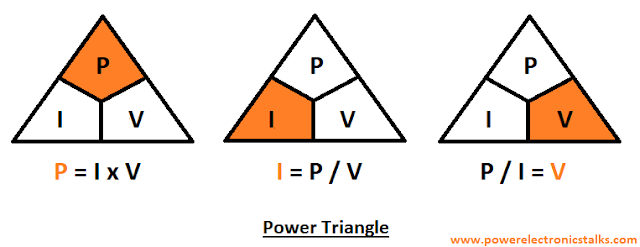Power Triangle

### Ohm’s law wheel

Now we know very well all the formulas related to ohm’s law formula and electric power formula.

By using these formulas, we can design ohms law wheel. According to Voltage, Current, Power, and Resistance twelve different formulas are mentioned in ohms law wheel.

Also, on the basis of same we can create Ohm's law Table of Formulas;

### Conclusion

The person who mostly deals with electrical and electronics terms or mostly with resistance the Ohm's law is an important rule to solve the current, voltage, resistance and power issues. The calculations presented here surely will help to calculate Ohm's law manually and to understand the Ohm's law concept. The calculator presented here will ease the calculation process.

## Resistor for LED Calculator

In almost all applications where LED’s are used, a resistor in series with LED is connected. Selection of resistor for LED is not a difficult task only step wise process has to follow which is given in this article.Resistor for LED Calculator
Also, Resistor for LED Calculator has been provided which will help you to calculate the LED resistor in easy way. Also, you can cross check your calculated LED resistor value is matching with the value generated by LED resistor calculator.

This calculator for LED resistor is very useful and easy to use.

### Click below button to get Resistor for LED Calculator or LED Resistor CalculatorResistor for LED Calculator
Now let discuss the steps to calculate Resistor for LED and same steps has been used to develop Resistor for LED Calculator;

### Use of Resistor for LED’s

LEDs are more common for almost many of applications as an indicator or light. This is because it has good power efficiency. Also, it has good life span as compare to incandescent or fluorescent lamps.

Full form of LED is Light Emitting Diode. It’s a type of diode. Like diode it also has polarity i.e. anode and cathode. With suitable application of power across it i.e. the power matching to LED specifications, LED works and produce light.

We have to follow the polarity requirement of the LED, same like diode otherwise it will fail. Because LED allows low value of reverse polarity voltage approximately 5 volts.

Know you know that LED is a diode so, the current across the LED should not cross the limit otherwise it will cause failure to the LED.

This is the reason why resistor in the series with the LED is used. In simple words the LED resistor controls the current across the LED.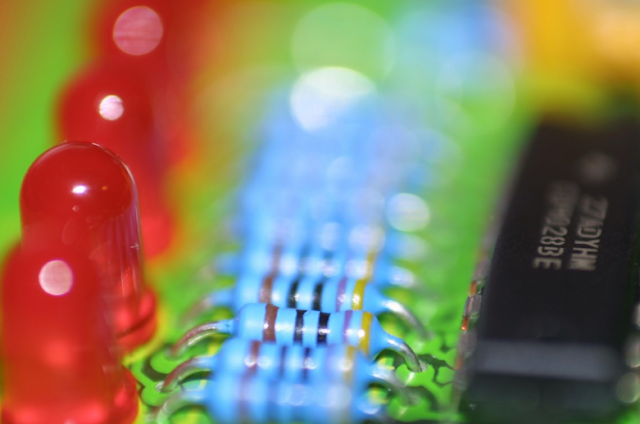Resistor with LED
Now let see the necessities or requirements for the current control resistor that must be used with a LED;

We know that LED’s are available in different colours. Each colour LED is made by different materials and these material have different voltage requirements. So, each colour LED has different electrical requirements and hence different specifications.

In LED specifications we refer a term, “Forward Voltage (Vf)”. This is the voltage which makes LED to work.

Below are forward voltage requirements of some common LED’s;

• Red = approximately 1.7 volts
• Green = approximately 2.2 volts
• Orange = approximately 2.0 volts
• Yellow = approximately 2.1 volts
• White = approximately 3.2 volts
• Blue = approximately 3.2 volts
Now we can clearly see the variation in the forward voltage. By knowing the forward voltage, we have to pass current in proper amount across the LED. So, resistor in series with LED is used, where resistor controls the current according to forward voltage.

### Correct resistor for LED

Without using a resistor, if we apply direct DC voltage to a LED, it will work.

But if the applied voltage and current is not matching to the requirement of LED, the LED will glow dim or bright or may be it get heated and fails.

In simple words, less current across LED, the LED will glow dim. More current across LED, the LED will glow bright.

If current crosses the electrical requirement of LED, the LED will fail.

So, a resistor we have to connect in series with the LED whose current we want to control.

### Calculation for selection of LED resistor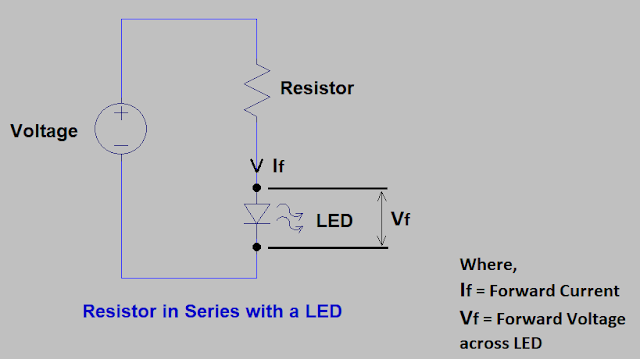Resistor in series with LED
The resistor selection depends on Ohm’s law i.e. R = V/I.

V is the applied DC voltage. This can be calculated as; V = (applied Vdc) – Vf.

I is the amount of current which should flow across the LED to make it ON.

Applied DC voltage should me more than forward voltage (Vf) of LED. i.e. if you apply 12Vdc and LED has Vf of 3.2V so, a drop of 3.2V is easily possible across the LED and it works. But less than 3.2 will never make LED to glow.

The typical forward voltage and forward current requirement of LED you can easily find in LED specification sheet.

Now our formula becomes;

R = (Vdc – Vf) / If

Now let try with an example;

Vdc = 12V

Vf = 3.2V

If = 0.010A

Then,

R = (12 – 3.2) / 0.010

R = 880 ohm. Select standard resistor value; R = 910 ohm.

Now calculate the power rating of resistor.

P = V x I

P = (12 – 3.2) x 0.010

P = 0.088W

We can select 0.25W for this application as it is more than the calculated one. If we use the wattage rating less then calculated value, the resistor become hot.

While creating "Resistor for LED Calculator" we followed same formulas.

### Many LED’s in a circuit

Consider a case, there’s a requirement of many LED’s in an application.

We can use multiple LED’s of same colour or different colour in parallel connection.

By this same voltage from voltage source will be available at each LED string, but the current requirement will increase according to number of LED’s.

As current increase the wattage requirement of each resistor will also increase.

Let understand this by an example;

Vdc = 12V

Vf = 3.2V

Number of LED strings in parallel = 5

Single LED current requirement = 0.010A

Total current requirement, I =5 x 0.010 = 0.050A

Using the formula;

R = (12 – 3.2)/0.050

R = 176 ohm. Select standard resistor value; R = 180 ohm.

Wattage we can calculate;

P = V x I = (12 – 3.2) x 0.050 = 0.44

We can select 0.25W for this application as it is more than the calculated value.

For different colour LED’s we have to calculate current requirement of each string and add all current.

In this case also voltage will be same and we have to subtract forward voltage from supply voltage.

Calculation of power rating of resistor value will remain same like previous performed calculations. As mentioned before you have to select high wattage resistor as compare to calculated one.

In "Resistor for LED Calculator" we had already included the calculator for both resistor for LED in series and resistor for LED in parallel.

"Resistor and Working of Resistor"

"Electrical Resistance and Resistivity"

"Standard Resistor Values"

### Conclusion

We had seen that the selection of resistor for LED is simple and we have to follow the Ohm’s law. But we have to give importance to LED’s specification sheet before performing any calculations. So, that the voltage, current and wattage should be in the limit and do not damage the resistor. The Resistor for LED Calculator will help you to easily calculate these values.Author & Editor

Hi, welcome to my blog, “Power Electronics Talks”.

I am an Electronics Engineer. I am passionate about Power Electronics and latest Technology. By profession I am design and application engineer and play with circuits.

Here at Power Electronics Talks, I write about all the stuffs related to Power Electronics. To share my knowledge I hold the hand of my blog, "www.powerelectronicstalks.com", where I can share all the technical details of Power Electronics and latest technical trends.